# cubic

## Exponentially many perfect matchings in cubic graphs ★★★

Author(s): Lovasz; Plummer

Conjecture   There exists a fixed constantso that every-vertex cubic graph without a cut-edge has at least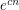perfect matchings.

Keywords: cubic; perfect matching

## Bigger cycles in cubic graphs ★★

Author(s):

Problem   Letbe a cyclically 4-edge-connected cubic graph and letbe a cycle of. Must there exist a cycleso that?

Keywords: cubic; cycle

## The intersection of two perfect matchings ★★

Author(s): Macajova; Skoviera

Conjecture   Every bridgeless cubic graph has two perfect matchings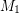,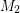so thatdoes not contain an odd edge-cut.

Keywords: cubic; nowhere-zero flow; perfect matching

## Barnette's Conjecture ★★★

Author(s): Barnette

Conjecture   Every 3-connected cubic planar bipartite graph is Hamiltonian.

Keywords: bipartite; cubic; hamiltonian

## Pentagon problem ★★★

Author(s): Nesetril

Question   Letbe a 3-regular graph that contains no cycle of length shorter than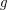. Is it true that for large enough~there is a homomorphism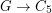?

Keywords: cubic; homomorphism

## Petersen coloring conjecture ★★★

Author(s): Jaeger

Conjecture   Letbe a cubic graph with no bridge. Then there is a coloring of the edges ofusing the edges of the Petersen graph so that any three mutually adjacent edges ofmap to three mutually adjancent edges in the Petersen graph.

Keywords: cubic; edge-coloring; Petersen graph

## The Berge-Fulkerson conjecture ★★★★

Author(s): Berge; Fulkerson

Conjecture   Ifis a bridgeless cubic graph, then there exist 6 perfect matchingsofwith the property that every edge ofis contained in exactly two of.

Keywords: cubic; perfect matching

## 5-flow conjecture ★★★★

Author(s): Tutte

Conjecture   Every bridgeless graph has a nowhere-zero 5-flow.

Keywords: cubic; nowhere-zero flow